Summation (Sigma) Notation MathBitsNotebook.com Terms of Use   Contact Person: Donna Roberts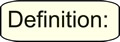A sequence (or progression) is an ordered list of numbers (values).

Sequence: 2, 4, 6, 8 10, 12, 14
The numbers (or values) are called "terms" or "elements" of the sequence.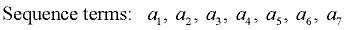A series is the sum you get when you add all of the terms of a sequence.

Series: 2 + 4 + 6 + 8 + 10 + 12 + 14 = 56

When writing a series, a shorthand notation is used to represent the sum of a number of terms having a common form. This shorthand notation is referred to as "summation notation" or "sigma notation". The symbol used in the notation, , is the Greek capital letter sigma.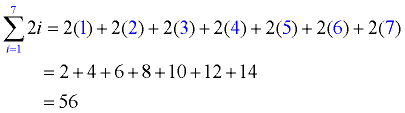Numbers are substituted into the expression (2i) starting with the first "i" value of "1" and continuing (counting by ones) until the "i" value reaches "7". All of these substitutions are then added, creating a series.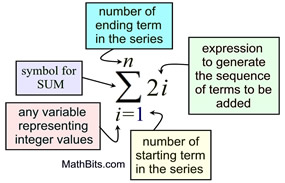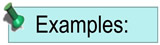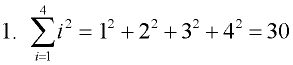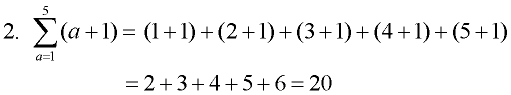Note: parentheses are used when the value representing the sequence consists of more than one term.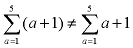because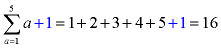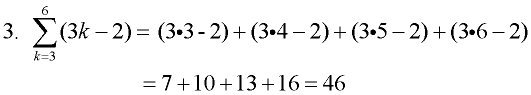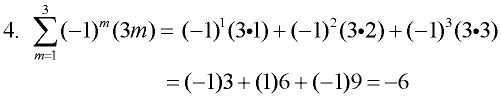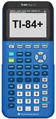For calculator help with summation notation click here.